地球物理学报2015, Vol. 58Issue (10): 3639-3648PDF

Mechanism of high frequency coupling instability and stable implementation for transmitting boundary —SH wave motion
ZHANG Xu-Bin, LIAO Zhen-Peng, XIE Zhi-Nan
Institute of Engineering Mechanics, China Earthquake Administration, Key Laboratory of Earthquake Engineering and Engineering Vibration of China Earthquake Administration, Harbin 150080, China
Abstract: Stable implementation of high order artificial boundary conditions has not been solved satisfactorily for wave simulation in infinite domain. The instability problem is studied for the multi-transmitting formula, one of the promising boundary schemes. Focusing on the lumped-mass finite element simulation of SH wave motion, mechanism of high frequency instability caused by coupling the formula and the finite element scheme is further clarified based on the group velocity interpretation of GKS theorem. The mechanism is that both the formula and the finite element scheme support out-going high frequency waves whose group velocity points to interior domain, thus wave energy is allowed to enter spontaneously from the boundary into interior domain. Such type of waves do not exist in the corresponding continuous model, it is solely induced by the finite element. A method for destroying the coupling is presented by changing the interior scheme via modifying the stiffness matrix of FEM using proper integration rule, meanwhile the accuracy of the modified scheme is no less than that of the original one. Theoretical analysis and numerical experiments have shown this method can ensure MTF to be implemented stably. The research approach developed in this study can be applied to cope with the boundary instable problems for other types of wave simulation, and the research results have application prospect for forward and inverse problems in geophysics.
Key words: Coupling instability     GKS theorem     Group velocity interpretation     Abnormal dispersion     Modified integration rules
1 引言

2 有限元离散格式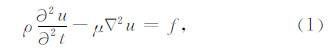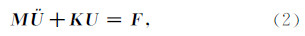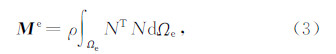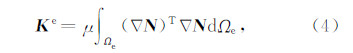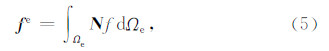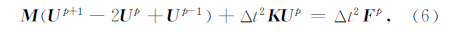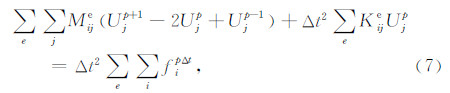图 1 长方形单元节点编号和二维局部节点系Fig. 1 Node number and 2D local grid system for rectangle element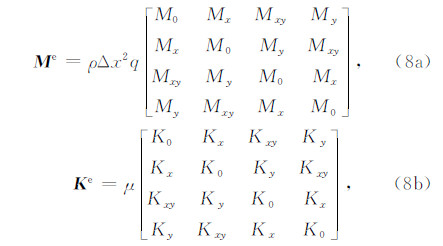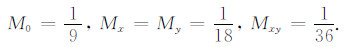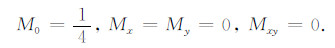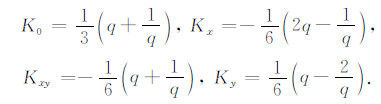ABC采用MTF(Liao et al.，1984a)，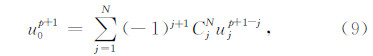3 高频耦合失稳机理

GKS定理的群速度解释表明，若内域离散格式和人工边界支持群速度指向内域的外行平面谐波，则将导致失稳.内域离散格式支持的平面谐波由频散分析可知，而对于人工边界支持的平面谐波，可将人工边界进行无限延拓当成内域离散格式进行频散分析，本文将无限延拓的MTF称之为MTF格式.因此，若内域与人工边界格式频散曲线的交点对应群速度向内的外行平面谐波，则导致耦合失稳.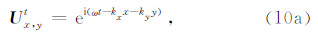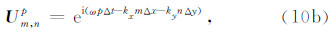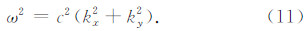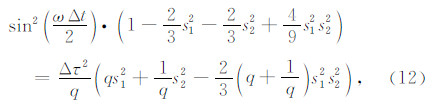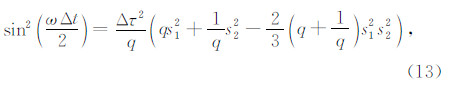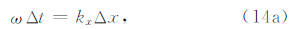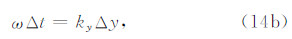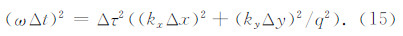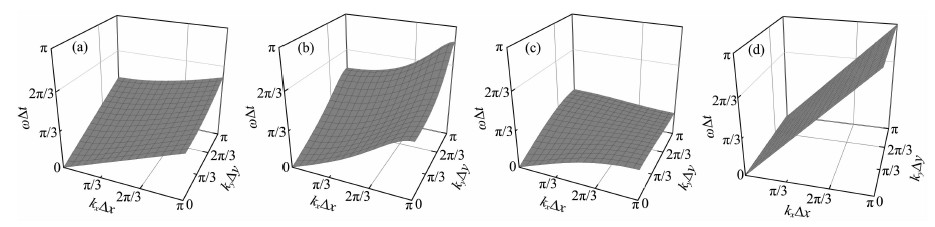图 2 q=1，Δτ=0.4时，连续模型和离散格式在第一象限的频散 (a)连续模型;(b)一致质量有限元;(c)集中质量有限元;(d)人工边界垂直 x 方向时的MTF格式.Fig. 2 The dispersion of continuous model and discrete schemes at the first quadrant when q=1，Δτ=0.4. (a)Continuous model;(b)Consistent mass FEM;(c)Lumped mass FEM;(d)MTF scheme when ABC is perpendicular to x-axis.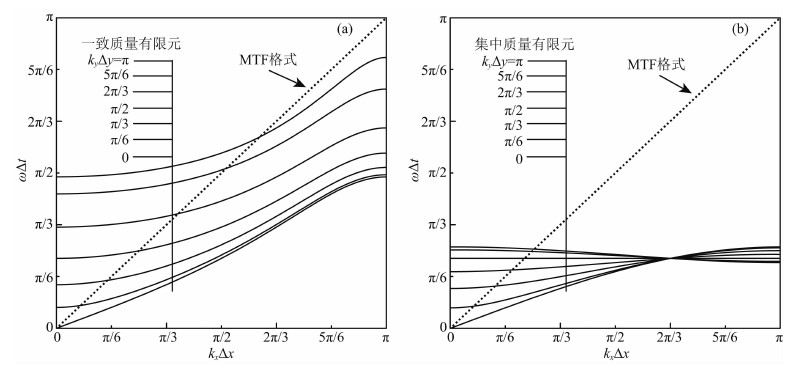图 3 q=1，Δτ=0.4时，在给定 kyΔy 不同取值下，离散格式在第一象限的频散曲线(a)一致质量有限元(实线)与人工边界垂直 x 方向时的MTF格式(虚线)的频散曲线(b)集中质量有限元(实线)与人工边界垂直 x 方向时的MTF格式(虚线)的频散曲线.Fig. 3 The dispersion curves of discrete schemes at the first quadrant under the given different value of kyΔy when q=1，Δτ=0.4(a)The dispersion curves of consistent mass FEM(solid lines) and MTF scheme(dash line)when ABC is perpendicular to x-axis;(b)The dispersion curves of lumped mass FEM(solid lines) and MTF scheme(dash line)when ABC is perpendicular to x-axis.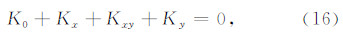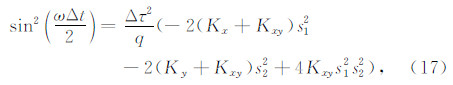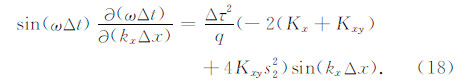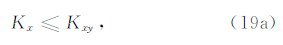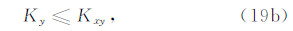4 修正的集中质量有限元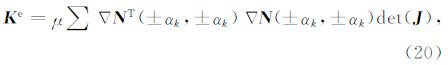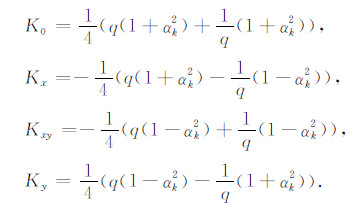αk=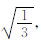，对应的有限元为之前讨论的集中质量有限元.以示区别，将之前讨论的称为传统的集中质量有限元，此处引入的称为修正的集中质量有限元.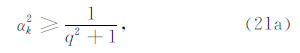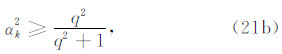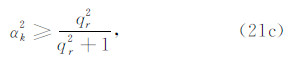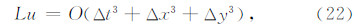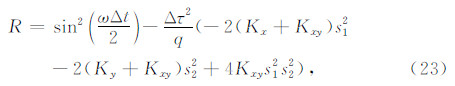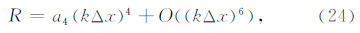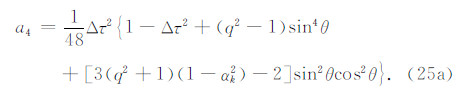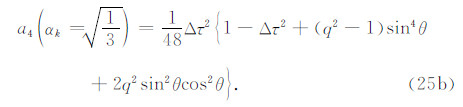q≥1 时，并由下文稳定性条件(30)式可知 Δτ≤1，故式(25a)和(25b)的共同项 1－Δτ2+(q2－1)sin4θ≥0. 若对于两式中的 sin2θcos2θ 项系数有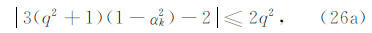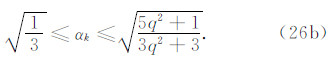q≥1可知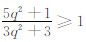，又有αk∈[0，1]，故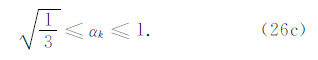q<1 时，先利用(sin2θ+cos2θ)2=1 改写式(25a)，即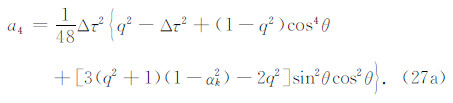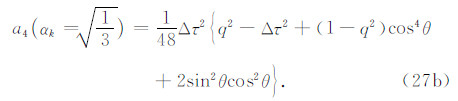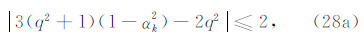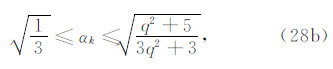q≤1 可知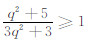，又有αk∈[0，1]，故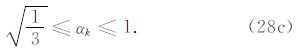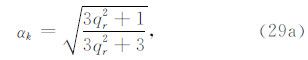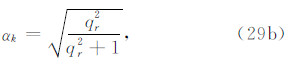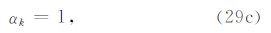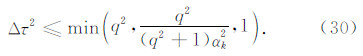5 数值实验

Liao等(1984a)中的算例由于计算时间不长，未出现MTF与传统有限元耦合所致的高频失稳.为了揭示MTF的高频数值失稳现象，以及验证MTF结合修正有限元可以稳定实现，本文数值实验采用Liao等(1984a)的均匀弹性半空间模型.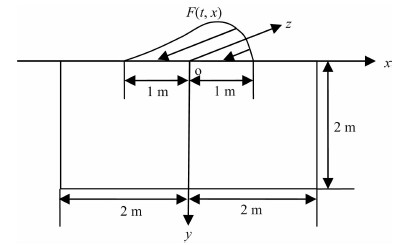图 4 弹性半空间SH波动模型Fig. 4 Elastic half-space model of SH wave motion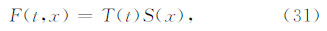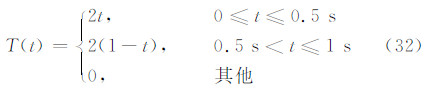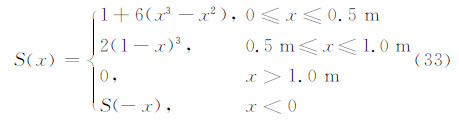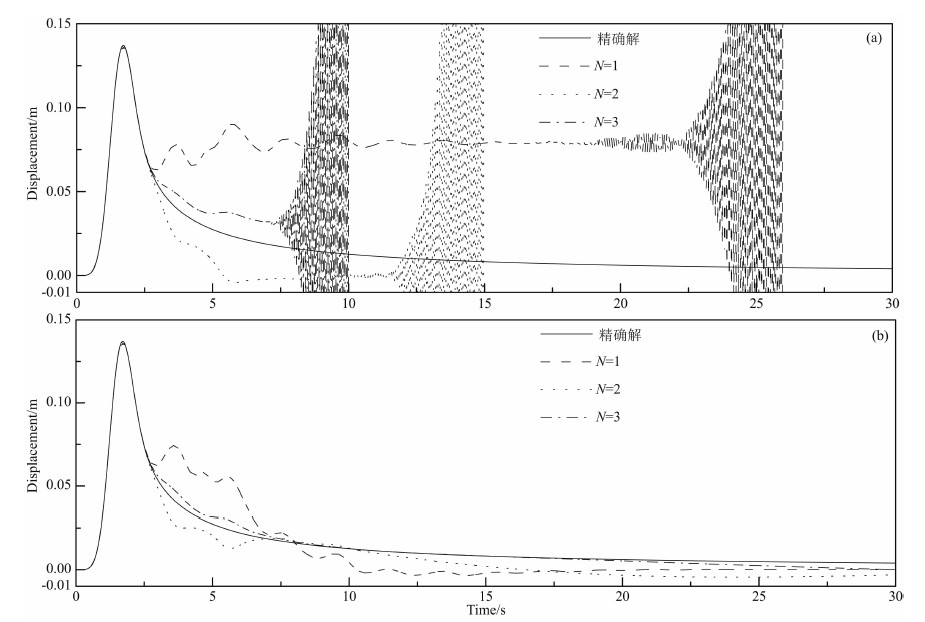图 5(a)和(b)分别为采用传统有限元和修正有限元结合MTF(式(9)，N=1，2，3)计算的观测点(1，0)位移时程离散方案为Δx=0.05 m，Δy=0.025 m，Δt=0.02 sFig. 5(a) and (b)are the displacement time history of the observation point(1，0)computed by classic FEM and modified FEM with MTF(formula(9)，N=1，2，3)separately. The discretization mesh is Δx=0.05 m，Δy=0.025 m，Δt=0.02 s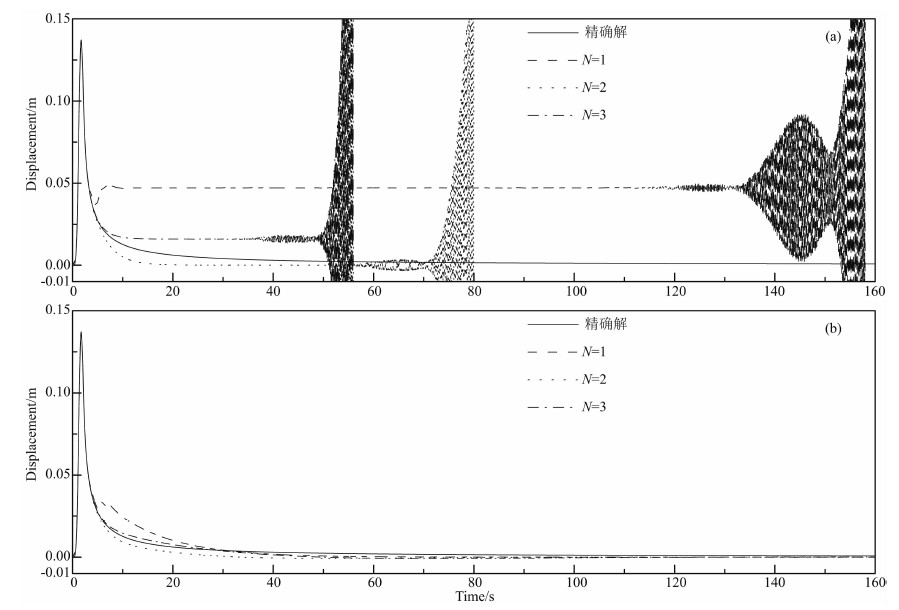图 6(a)和(b)分别为采用传统有限元和修正有限元结合MTF(空间内插公式，人工波速 ca=c，N=1，2，3) 计算的观测点(1，0)位移时程，离散方案为Δx=Δy=0.05 m，Δt=0.025 sFig. 6(a) and (b)are the displacement time history of the observation point(1，0)computed by classic FEM and modified FEM with MTF(spatial interpolation formula with artificial wave velocity ca=c，N=1，2，3)separately. The discretization mesh is Δx=Δy=0.05 m，Δt=0.025 s
6 结论

  Baffet D,Bielak J,Givoli D,et al. 2012. Long-time stable high-order absorbing boundary conditions for elastodynamics. Computer Methods in Applied Mechanics and Engineering,241-244: 20-37.  Bérenger J P. 1994. A perfectly matched layer for the absorption of electromagnetic waves. Journal of Computational Physics,114(2): 185-200.  Chew W C,Weedon W H. 1994. A 3-D perfectly matched medium from modified Maxwell's equations with stretched coordinates. Microwave Opt. Technol. Lett.,7(13): 599-604.  Clayton R,Engquist B. 1977. Absorbing boundary conditions for acoustic and elastic wave equations. Bull. Seism. Soc. Am.,67(6): 1529-1540.  Deeks A J,Randolph M F. 1994. Axisymmetric time-domain transmitting boundaries. Journal of Engineering Mechanics,120(1): 25-42.  Du X L,Zhao M,Wang J T. 2006. A stress artificial boundary in FEA for near-field wave problem. Chinese Journal of Theoretical and Applied Mechanics (in Chinese),38(1): 49-56.  Du X L,Zhao M. 2010. A local time-domain transmitting boundary for simulating cylindrical elastic wave propagation in infinite media. Soil Dynamics and Earthquake Engineering,30(10): 937-946.  Givoli D,Neta B. 2003. High-order non-reflecting boundary scheme for time dependent waves. J. Comput. Phys.,186(1): 24-46.  Gustafsson B,Kreiss H O,Sundström A. 1972. Stability theory of difference approximations for mixed initial boundary value problems II. Mathematics of Computation,26(119): 649-686.  Hagstrom T,Warburton T. 2004. A new auxiliary variable formulation of high-order local radiation boundary conditions: corner compatibility conditions and extensions to first order systems. Wave Motion,39(4): 327-338.  Higdon R L. 1986. Absorbing boundary conditions for difference approximations to the multi-dimensional wave equation. Mathematics of Computation,47(176): 437-459.  Higdon R L. 1990. Radiation boundary conditions for elastic wave propagation. SIAM Journal on Numerical Analysis,27(4): 831-869.  Idesman A,Pham D. 2014. Finite element modeling of linear elastodynamics problems with explicit time-integration methods and linear elements with the reduced dispersion error. Computer Methods in Applied Mechanics and Engineering,271: 86-108.  Jing L P,Liao Z P,Zou J X. 2002. A high-frequency instability mechanism in numerical realization of multi-transmitting formula. Earthquake Engineering and Engineering Vibration (in Chinese),22(1): 7-13.  Liao Z P,Wong H L,Yang B P,et al. 1984a. A transmitting boundary for transient wave analyses. Scientia Sinica (Series A),27(10): 1063-1076.  Liao Z P,Wong H L. 1984b. A transmitting boundary for the numerical simulation of elastic wave propagation. International Journal of Soil Dynamics and Earthquake Engineering,3(4): 174-183.  Liao Z P,Liu J B. 1992. Numerical instabilities of a local transmitting boundary. Earthquake Engineering and Structural Dynamics,21(1): 65-77.  Liao Z P,Xie Z N. 2011. Stability of numerical simulation of wave motion. Journal of Harbin Engineering University (in Chinese),32(9): 1254-1261.  Liao Z P. 2002. Introduction to Wave Motion Theories in Engineering (Second edition) (in Chinese). Beijing: Science Press.  Liao Z P,Zhou Z H,Zhang Y H. 2002. Stable implementation of transmitting boundary in numerical simulation of wave motion. Chinese Journal of Geophysics (in Chinese),45(4): 554-568,doi: 10.3321/j.issn:0001-5733.2002.04.011.  Li X J,Liao Z P. 1996. The drift instability of local transmitting boundary in time domain. Acta Mechanica Sinica (in Chinese),28(5): 627-632.  Li X J,Yang Y. 2012. Measures for stability control of transmitting boundary. Chinese Journal of Geotechnical Engineering (in Chinese),34(4): 641-645.  Liu J B,Wang Z Y,Du X L,et al. 2005. Three-dimensional visco-elastic artificial boundaries in time domain for wave motion problems. Engineering Mechanics (in Chinese),22(6): 46-51.  Lysmer J,Kulemeyer R L. 1969. Finite dynamic model for infinite media. Journal of Engineering Mechanics Division,95(4): 859-878.  Trefethen L N. 1983. Group velocity interpretation of the stability theory of Gustafsson Kreiss and Sundström. Journal of Computational Physics,49(2): 199-217.  Xie Z N,Komatitsch D,Martin R,et al. 2014. Improved forward wave propagation and adjoint-based sensitivity kernel calculations using a numerically stable finite-element PML. Geophys. J. Int.,198(3): 1714-1747.  Xie Z N,Liao Z P. 2008. A note for the mechanism of high-frequency oscillation instability resulted from absorbing boundary conditions. Acta Seismologica Sinica,21(3): 306-310.  Xie Z N,Liao Z P. 2012. Mechanism of high frequency instability caused by transmitting boundary and method of its elimination—SH wave. Chinese Journal of Theoretical and Applied Mechanics (in Chinese),44(4): 745-752.  Yue B,Guddati M N. 2005. Dispersion-reducing finite elements for transient acoustics. J. Acoust. Soc. Am.,118(4): 2132-2141.  Zhou Z H,Liao Z P. 2001. A measure for eliminating drift instability of the multi-transmitting formula. Acta Mechanica Sinica (in Chinese),33(4): 550-554.  杜修力,赵密,王进廷. 2006. 近场波动模拟的人工应力边界条件. 力学学报,38(1): 49-56.  景立平,廖振鹏,邹经湘. 2002. 多次透射公式的一种高频失稳机制. 地震工程与工程振动,22(1): 7-13.  廖振鹏. 2002. 工程波动理论导论(第二版). 北京: 科学出版社.  廖振鹏,周正华,张艳红. 2002. 波动数值模拟中透射边界的稳定实现. 地球物理学报,45(4): 554-568,doi: 10.3321/j.issn:0001-5733.2002.04.011.  廖振鹏,谢志南. 2011. 波动数值模拟的稳定性. 哈尔滨工程大学学报,32(9): 1254-1261.  李小军,廖振鹏. 1996. 时域局部透射边界的计算飘移失稳. 力学学报,28(5): 627-632.  李小军,杨宇. 2012. 透射边界稳定性控制措施探讨. 岩土工程学报,34(4): 641-645.  刘晶波,王振宇,杜修力等. 2005. 波动问题中的三维时域粘弹性人工边界. 工程力学,22(6): 46-51.  谢志南,廖振鹏. 2012. 透射边界高频失稳机理及其消除方法–SH波动. 力学学报,44(4): 745-752.  周正华,廖振鹏. 2001. 消除多次透射公式飘移失稳的措施. 力学学报,33(4): 550-554.